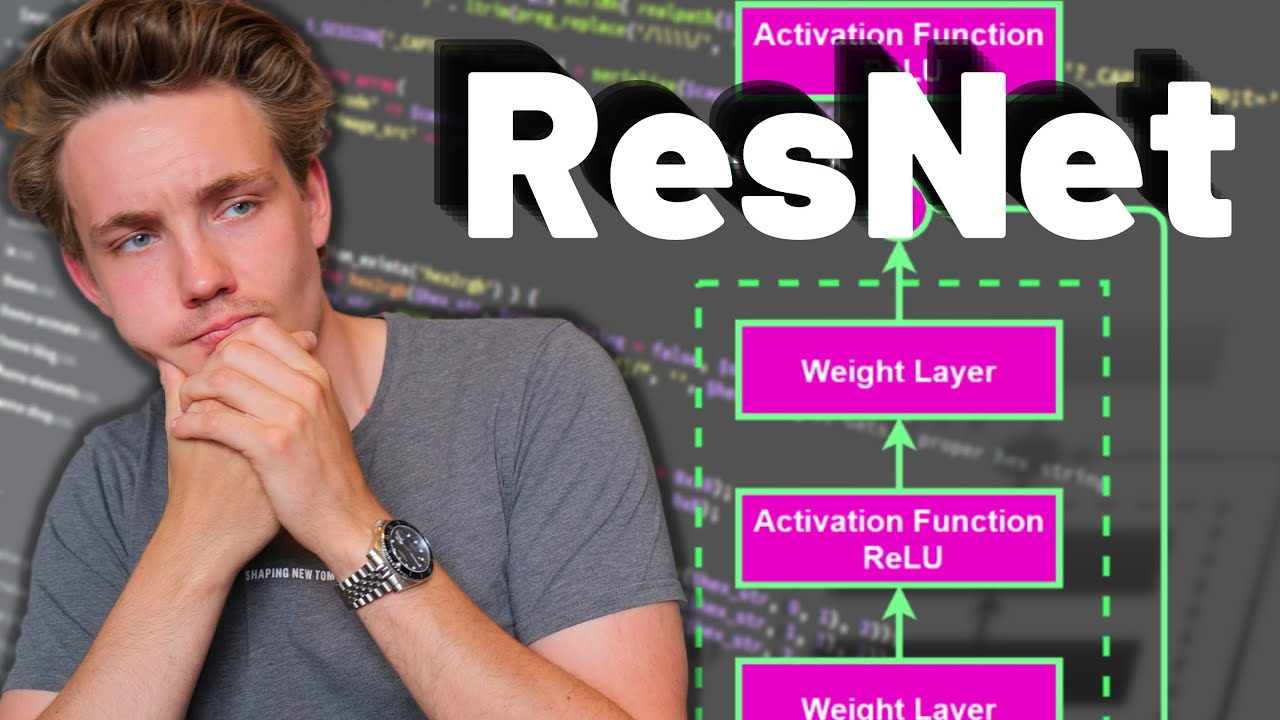# ResNet Architecture and Residual Block Explained - Neural Networks and Deep LearningIn this Neural Networks and Deep Learning Tutorial, we will talk about the ResNet Architecture. Residual Neural Networks are often used to solve computer vis...

In this Neural Networks and Deep Learning Tutorial, we will talk about the ResNet Architecture. Residual Neural Networks are often used to solve computer vision problems and consist of several residual blocks. We will walk about what a residual block is and compare it to the architecture of a standard convolutional neural network. I'll show you how you can use the pre-trained ResNets from Keras and TensorFlow.

## TensorFlow And Keras Tutorial | Deep Learning With TensorFlow & Keras | Deep Learning

This video on TensorFlow and Keras tutorial will help you understand Deep Learning frameworks, what is TensorFlow, TensorFlow features and applications, how TensorFlow works, TensorFlow 1.0 vs TensorFlow 2.0, TensorFlow architecture with a demo. Then we will move into understanding what is Keras, models offered in Keras, what are neural networks and they work.

## Top Deep Learning Development Services | Hire Deep Learning Developer

Inexture's Deep learning Development Services helps companies to develop Data driven products and solutions. Hire our deep learning developers today to build application that learn and adapt with time.

## Pytorch vs Tensorflow vs Keras | Deep Learning Tutorial (Tensorflow, Keras & Python)

We will go over what is the difference between pytorch, tensorflow and keras in this video. Pytorch and Tensorflow are two most popular deep learning frameworks. Pytorch is by facebook and Tensorflow is by Google. Keras is not a full fledge deep learning framework, it is just a wrapper around Tensorflow that provides some convenient APIs.

## Why Deep Learning is Becoming so Popular? | Deep Learning Tutorial (TensorFlow 2.0, Keras & Python)

This video explains four reasons why deep learning has become so popular in past few years. In this deep learning tutorial python, I will cover following things in this video: Introduction; Data growth; Hardware advancements; Python and opensource ecosystem; Cloud and AI boom

## Keras with TensorFlow Course - Python Deep Learning and Neural Networks

Keras with TensorFlow Course - Python Deep Learning and Neural Networks for Beginners Tutorial: How to use Keras, a neural network API written in Python and integrated with TensorFlow. We will learn how to prepare and process data for artificial neural networks, build and train artificial neural networks from scratch, build and train convolutional neural networks (CNNs), implement fine-tuning and transfer learning, and more!